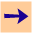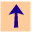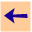9 Assumables

# 9 Assumables

Assumables exist as in Chapter 9 of Computational Intelligence. There are no integrity constraints that are handled specially. The interaction between assumables and negation as failure is unsatisfactory, in that `~`g succeeds if there is provably no explanation of g.

To to make an atom assumable, you can issue the command:

```cilog: assumable atom.
```
When the atom is encountered, it is assumed to be true. The assumptions used to make a proof go though are collected and presented to the user when the answer is returned.
```cilog: tell a(X) <- b(X) & c(X).
cilog: assumable c(X).
cilog: tell b(X) <- d(X) & e(X).
cilog: tell d(a).
cilog: tell d(b).
cilog: tell d(c).
Is e(a) true? [yes,no,unknown,why,help]: yes.
Assuming: [c(a)].
[more,ok,how,help]: more.
Is e(b) true? [yes,no,unknown,why,help]: no.
Is e(c) true? [yes,no,unknown,why,help]: yes.9 Assumables